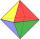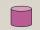# Hexagonal prism 2

The regular hexagonal prism has a surface of 140 cm2 and height of 5 cm. Calculate its volume.

Result

V =  20.175 cm3

#### Solution:Checkout calculation with our calculator of quadratic equations.

Leave us a comment of example and its solution (i.e. if it is still somewhat unclear...):Be the first to comment!## Next similar examples:

1. OctahedronAll walls of regular octahedron are identical equilateral triangles. ABCDEF octahedron edges have a length d = 6 cm. Calculate the surface area and volume of this octahedron.
2. Cylinder diameterThe surface of the cylinder is 149 cm2. The cylinder height is 6 cm. What is the diameter of this cylinder?
3. RootsDetermine the quadratic equation absolute coefficient q, that the equation has a real double root and the root x calculate: ?
4. RectangleCalculate area of the rectangle if its length is 12 cm longer than its width and length is equal to the square of its width.
5. Expression with powersIf x-1/x=5, find the value of x4+1/x4Find the roots of the quadratic equation: 3x2-4x + (-4) = 0.
7. EquationEquation ? has one root x1 = 8. Determine the coefficient b and the second root x2.
8. AlgebraX+y=5, find xy (find the product of x and y if x+y = 5)
9. DiscriminantDetermine the discriminant of the equation: ?
10. Evaluation of expressionsIf a2-3a+1=0, find (i)a2+1/a2 (ii) a3+1/a3
11. ProductThe product of two consecutive odd numbers is 8463. What are this numbers?
12. Perimeter of RTFind the circumference of the rectangular triangle if the sum of its legs is 22.5 cm and its area is 62.5 cm2.Solve quadratic equation: 2x2-58x+396=0Children's tickets to the swimming pool stands x € for an adult is € 2 more expensive. There was m children in the swimming pool and adults three times less. How many euros make treasurer for pool entry?We want to prove the sentence: If the natural number n is divisible by six, then n is divisible by three. From what assumption we started?The farmer would like to first seed his small field. The required amount depends on the seed area. Field has a triangular shape. The farmer had fenced field, so he knows the lengths of the sides: 119, 111 and 90 meters. Find a suitable way to determine thDetermine the coefficient of this sequence: 7.2; 2.4; 0.8